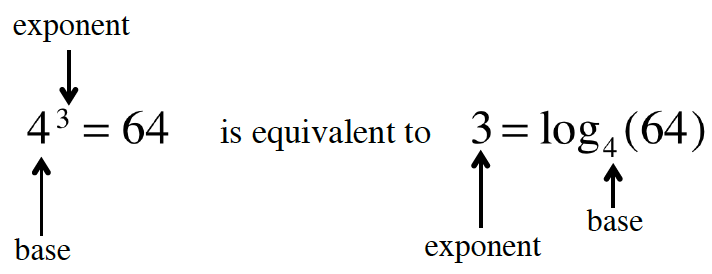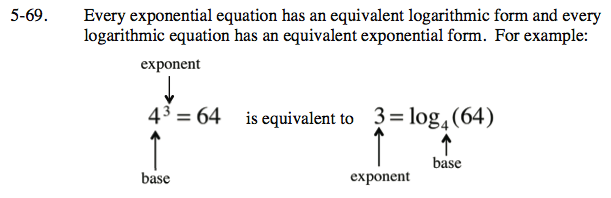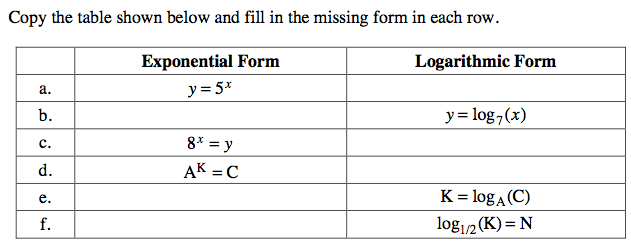### Home > INT3 > Chapter Ch5 > Lesson 5.2.2 > Problem5-69

5-69.
1. Every exponential equation has an equivalent logarithmic form and every logarithmic equation has an equivalent exponential form. For example: Homework Help ✎

2. ###3. Copy the table shown below and fill in the missing form in each row.

4.  Exponential Form Logarithmic Form a. y = 5x b. y = log7(x) c. 8x = y d. AK = C e. K = logA(C) f. log1/2(K) = N